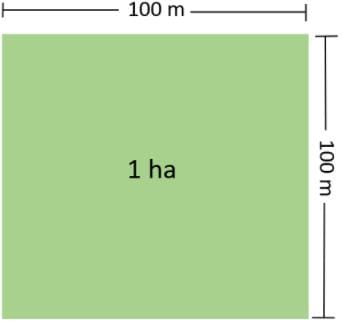UPSC  >  Test: Motion & Measurement of Distances

# Test: Motion & Measurement of Distances

Test Description

## 10 Questions MCQ Test Science & Technology for UPSC CSE | Test: Motion & Measurement of Distances

Test: Motion & Measurement of Distances for UPSC 2023 is part of Science & Technology for UPSC CSE preparation. The Test: Motion & Measurement of Distances questions and answers have been prepared according to the UPSC exam syllabus.The Test: Motion & Measurement of Distances MCQs are made for UPSC 2023 Exam. Find important definitions, questions, notes, meanings, examples, exercises, MCQs and online tests for Test: Motion & Measurement of Distances below.
Solutions of Test: Motion & Measurement of Distances questions in English are available as part of our Science & Technology for UPSC CSE for UPSC & Test: Motion & Measurement of Distances solutions in Hindi for Science & Technology for UPSC CSE course. Download more important topics, notes, lectures and mock test series for UPSC Exam by signing up for free. Attempt Test: Motion & Measurement of Distances | 10 questions in 10 minutes | Mock test for UPSC preparation | Free important questions MCQ to study Science & Technology for UPSC CSE for UPSC Exam | Download free PDF with solutions
 1 Crore+ students have signed up on EduRev. Have you?
Test: Motion & Measurement of Distances - Question 1

### The standard unit of length in SI system is

Detailed Solution for Test: Motion & Measurement of Distances - Question 1

The standard unit of length in SI system is metre.

Test: Motion & Measurement of Distances - Question 2

### What device should a tailor use to measure the length of cloth?

Detailed Solution for Test: Motion & Measurement of Distances - Question 2

Measuring tape is used by a tailor to measure the length of cloth.

Test: Motion & Measurement of Distances - Question 3

### One cm is equal to

Detailed Solution for Test: Motion & Measurement of Distances - Question 3

One centimetre (cm) is equal to:

► 10 mm
► 10-2m
► 10-5km

Test: Motion & Measurement of Distances - Question 4

The movement of earth around the sun is an example of

Detailed Solution for Test: Motion & Measurement of Distances - Question 4
• Oscillatory motion takes place when the restoring force acting on the body is directly proportional to its displacement from its equilibrium position. In practice, oscillatory motion eventually comes to rest due to damping or frictional forces.
• Non-periodic motion: The motion which does not repeat itself after a regular interval of time is called non-periodic motion. Examples: A footballer running on a field, application of brakes in a moving vehicle, a ball rolling the ground gradually slows down finally stops, the motion of tides in the sea, etc.
• A type of motion in which all parts of the body move the same distance in a given time is known as translatory motion. Translatory motion can be of two types: rectilinear and curvilinear.
• The movements of the earth around the sun are an example of periodic motion as it is completed in a fixed interval of time.
Test: Motion & Measurement of Distances - Question 5

The distance between Delhi and Kanpur is usually expressed in

Detailed Solution for Test: Motion & Measurement of Distances - Question 5

The distance between Delhi and Kanpur is usually expressed in kilometre.

Test: Motion & Measurement of Distances - Question 6

Which of the following does not represent a time-interval?

Detailed Solution for Test: Motion & Measurement of Distances - Question 6

Time of sunrise is a point of time while a day, a minute and a second represent a period of time or time interval.

Test: Motion & Measurement of Distances - Question 7

One kilometer is equal to

Detailed Solution for Test: Motion & Measurement of Distances - Question 7

One kilometre is equal to 1000 meter.

Test: Motion & Measurement of Distances - Question 8

Hectare is the unit of

Detailed Solution for Test: Motion & Measurement of Distances - Question 8

Hectare is the unit of area which is equal to 10000 meter square.Test: Motion & Measurement of Distances - Question 9

Which one is not a/an ancient unit of measurement?

Detailed Solution for Test: Motion & Measurement of Distances - Question 9

Girah, Cubit and Angul are ancient unit of measurement while meter is SI unit of measurement.

Test: Motion & Measurement of Distances - Question 10

Change in position of a body with time is called

Detailed Solution for Test: Motion & Measurement of Distances - Question 10

Change in position of a body with time is called motion.

## Science & Technology for UPSC CSE

86 videos|352 docs|235 tests
 Use Code STAYHOME200 and get INR 200 additional OFF Use Coupon Code
Information about Test: Motion & Measurement of Distances Page
In this test you can find the Exam questions for Test: Motion & Measurement of Distances solved & explained in the simplest way possible. Besides giving Questions and answers for Test: Motion & Measurement of Distances, EduRev gives you an ample number of Online tests for practice

## Science & Technology for UPSC CSE

86 videos|352 docs|235 tests

### How to Prepare for UPSC

Read our guide to prepare for UPSC which is created by Toppers & the best Teachers